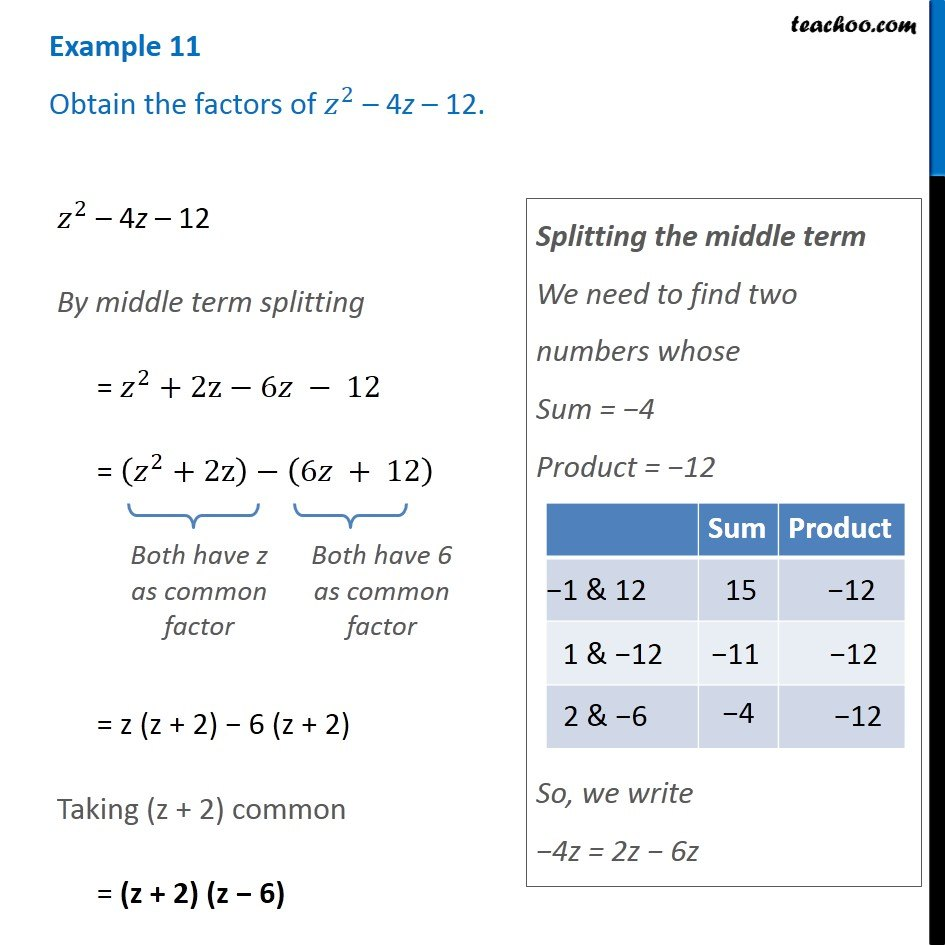Examples

Chapter 12 Class 8 Factorisation
Serial order wiseLearn in your speed, with individual attention - Teachoo Maths 1-on-1 Class

### Transcript

Example 11 Obtain the factors of 𝑧^2 – 4z – 12. 𝑧^2 – 4z – 12 By middle term splitting = 𝑧^2+"2z"−6𝑧 − 12 = (𝑧^2+"2z" )−(6𝑧 + 12) Both have z as common factor Both have 6 as common factor = z (z + 2) − 6 (z + 2) Taking (z + 2) common = (z + 2) (z − 6) Splitting the middle term We need to find two numbers whose Sum = −4 Product = −12 So, we write −4z = 2z − 6z